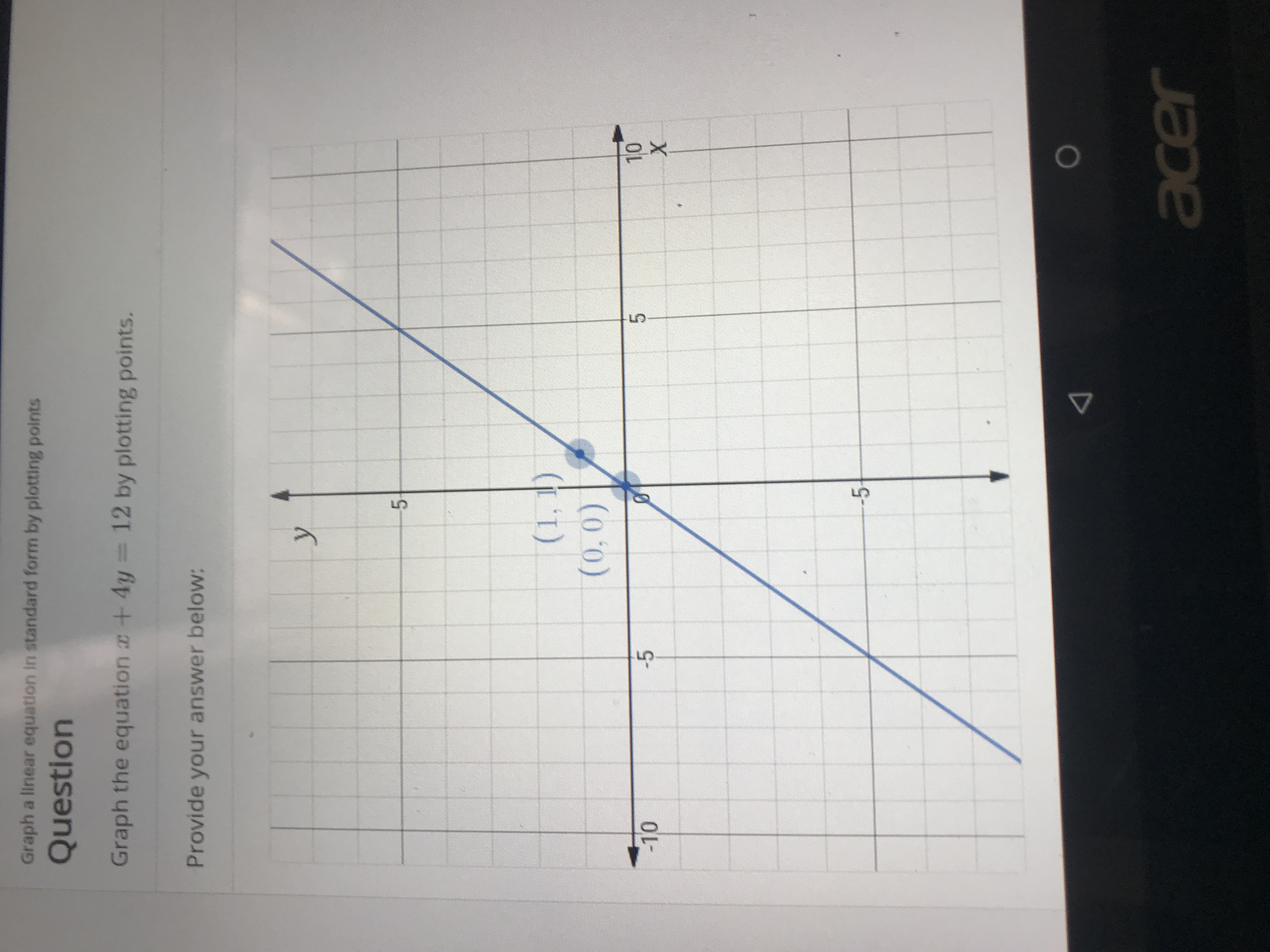# Graph a linear equation in standard form by plotting pointsQuestionGraph the equation +4y12 by plotting points.Provide your answer below:y5(1,1)(0,0)10X5-5-10-5acer

Question
22 viewshelp_outlineImage TranscriptioncloseGraph a linear equation in standard form by plotting points Question Graph the equation +4y 12 by plotting points. Provide your answer below: y 5 (1,1) (0,0) 10 X 5 -5 -10 -5 acer fullscreen
check_circle

Step 1

Lets make a table for x+4y=12

x=12-4y

Picked few random v...

### Want to see the full answer?

See Solution

#### Want to see this answer and more?

Solutions are written by subject experts who are available 24/7. Questions are typically answered within 1 hour.*

See Solution
*Response times may vary by subject and question.
Tagged in

### Algebra